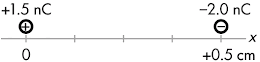## 20160303

### Physics quiz question: electric force on test charge

Physics 205B Quiz 3, spring semester 2015
Cuesta College, San Luis Obispo, CATwo point charges are held at fixed positions. A +1.5 nC charge is at the origin, and  a second –2.0 nC charge is at x = +0.5 cm. The force experienced by the –2.0 nC charge is directed to the __________, and its magnitude is __________.
(A) left; 5×10–6 N.
(B) left; 1×10–3 N.
(C) left; 5×105 N.
(D) right; 5×10–6 N.
(E) right; 1×10–3 N.
(F) right; 5×105 N.

Correct answer (highlight to unhide): (B)

The force exerted on the –2.0 nC charge at x = +0.5 cm points to the left, as it is being attracted by the +1.5 nC charge at the origin.

The magnitude of the force on the –2.0 nC charge at x = +0.5 cm is given by:

|F1 on 2| = k·|q1|·|q2|/(r2),

where q1 = +1.5 nC is the source charge at the origin, and q2 = –2.0 nC is the test charge (the charge that force is being exerted on) at x = +0.5 m, and the proportionality constant k = 8.99×109 N·m2/C2. Then:

|F1 on 2| = (8.99×109 N·m2/C2)·|+1.5×10–9 C|·|–2.0×10–9 C|/(0.5×10–2 m)2,

|F1 on 2| = 0.0010788 N,

or to one significant figure, the magnitude of the force on the –2.0 nC charge is 1×10–3 N.

(Response (A) has a magnitude of k·|q1|·|q2|/r; response (C) has a magnitude of k·|q1|/(r2). Responses (D)-(F) have the wrong force direction.)

Sections 30882, 30883
Exam code: quiz03wJnb
(A) : 11 students
(B) : 21 students
(C) : 4 students
(D) : 3 students
(E) : 4 students
(F) : 1 student

Success level: 48%
Discrimination index (Aubrecht & Aubrecht, 1983): 0.80